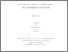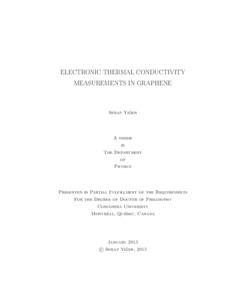Title:

# Electronic Thermal Conductivity Measurements in Graphene

Yiğen, Serap (2015) Electronic Thermal Conductivity Measurements in Graphene. PhD thesis, Concordia University.Preview
Text (application/pdf)
Yigen_PhD_S2015.pdf - Accepted Version
8MB

## Abstract

The electronic thermal conductivity in graphene describes how energy is transported by the charge carriers in graphene, and how these carriers lose their energy via diffusion and interactions with phonons and impurities. Understanding these interactions can shed light on electron-phonon scatterings, thermal relaxation processes, and the electron cooling mechanisms in graphene. We developed a method to experimentally isolate the electronic thermal conductivity in suspended graphene transistors by adapting a Joule self-heating method. We extracted the electronic thermal conductivity, $K_{e}$, as a function of electron temperature and charge carrier density.​
We fabricated two-point suspended graphene transistors using micro-fabrication methods. We used the electrical contacts as source and drain to apply a bias voltage and a back-gate electrode to tune the carrier density. We adapted a Joule self-heating method in which we used graphene as its own heater and thermometer. To do so, we prepared thermometry (calibration) curves by measuring low-bias resistance of the graphene devices versus temperature. As we increased the bias voltage, we could measure and control the temperature of electrons. We solved a one-dimensional heat diffusion equation and extracted the electronic thermal conductivity. We studied our samples at low bias voltages and intermediate temperatures where the electron and lattice temperatures are decoupled. This minimized the energy transfer between phonons and charge carriers. Since the suspended devices isolate the graphene crystals from the substrate, there were no interactions with the substrate phonons and no heat leakage to the substrate. Therefore, the heat was diffused only by the charge carriers.​
We extracted the electronic thermal conductivity in intrinsic monolayer graphene over a temperature range of 20 to 300 K. We found that $K_{e}$ has a strong temperature dependence, ranging from 0.5 to 11 W/m.K. We compared our data with a model of diffusing charged quasiparticles which have the same mean free path and velocity as graphene's charge carriers. Data from three different devices are in very good agreement with the model, supporting that the heat is carried by diffusing Dirac quasiparticles. ​
We doped our devices using the back-gate electrode, and extracted $K_{e}$ in doped graphene over a temperature range of 50 to 160 K. We found that $K_{e}$ is proportional to the charge conductivity times the temperature, and thus the Wiedemann-Franz Law is obeyed in suspended graphene. The Lorenz coefficient is estimated to be 1.1 to 1.7 $\times$ 10$^{-8}$ W $\Omega$ K$^{-2}$. We observed a strong thermal transistor effect in our devices as the charge carrier density is changed from $\approx$ 0.5 to 1.8 $\times$ 10$^{11}$ cm$^{-2}$, showing that $K_{e}$ can be tuned by more than a factor of 2 by applying a few volts of gate voltage. ​
The methods presented here could be extended to bilayer graphene devices and other two-dimensional materials to isolate $K_{e}$ to study electron-electron and electron-phonon interactions. The ability to control $K_{e}$ could be useful for energy harvesting in nano and opto-electronic devices.

Divisions: Concordia University > Faculty of Arts and Science > Physics Thesis (PhD) Yiğen, Serap Concordia University Ph. D. Physics April 2015 980061 SERAP YIGEN 16 Jul 2015 15:36 18 Jan 2018 17:50
All items in Spectrum are protected by copyright, with all rights reserved. The use of items is governed by Spectrum's terms of access.

Repository Staff Only: item control pageResearch related to the current document (at the CORE website)
Back to top